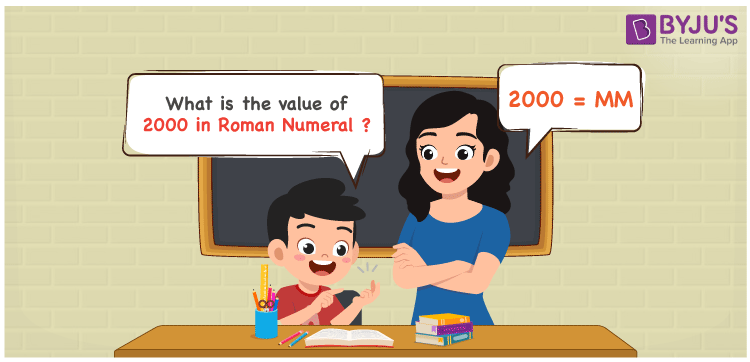# 2000 in Roman Numerals

2000 in Roman Numerals is MM. Any Roman numeral uses only the 7 primary symbols which are I, V, X, L, C, D, and M. Here, M represents 1000. MM represents the number 2000. What are Roman numerals? can be referred for more details. The details on how to write 2000 is given in the next section of this article.

 Number Roman Numeral 2000 MM

## How to Write 2000 in Roman Numerals?To convert 2000 in Roman Numerals, we need to represent 2000 as the sum of the fundamental symbols. 2000 can be broken down as the sum of 1000 and 1000. Since M is the symbol for 1000, 2000 will be MM.

2000 = 1000 + 1000

2000 = M + M

2000 = MM

## Video Lesson on Roman Numerals## Frequently Asked Questions on 2000 in Roman Numerals

Q1

### How to write the number 2000 in Roman Numerals?

2000 in Roman Numerals is written as MM.
Q2

### Does MM represent Roman Numerals?

Yes. MM represents Roman Numerals and it is the number 2000
Q3

### What is the year 2000 in Roman numerals?

The year 2000 in Roman Numerals is MM.